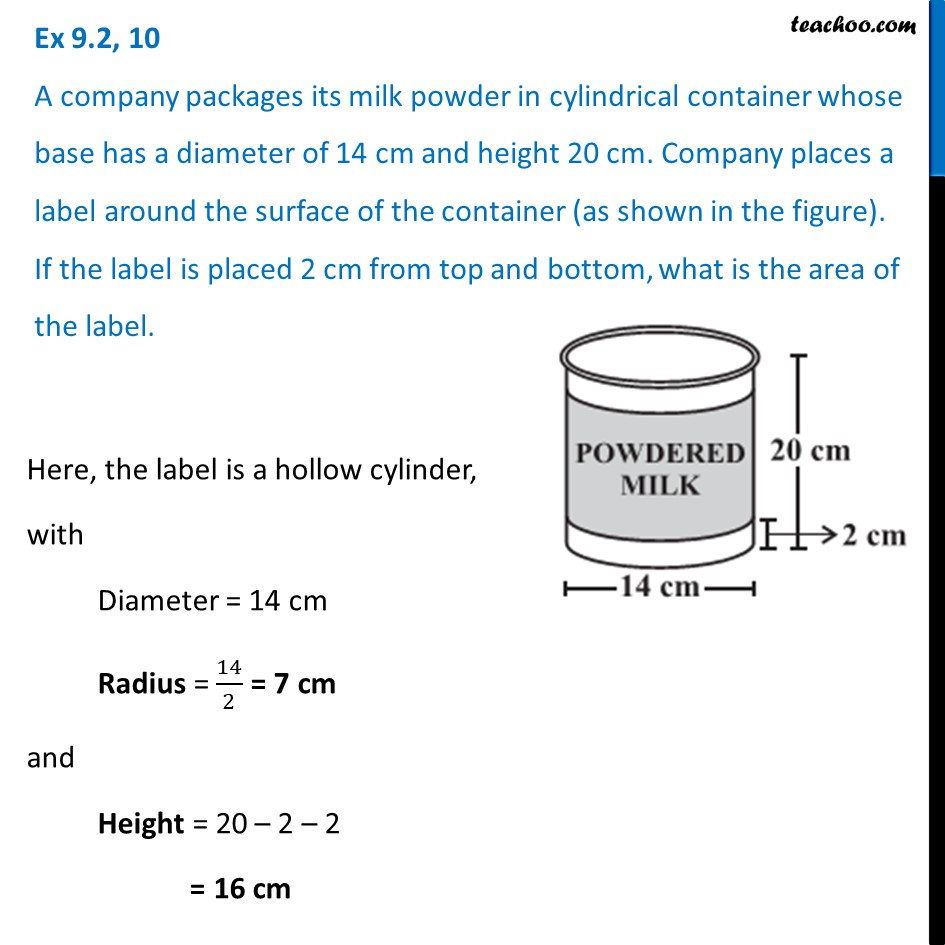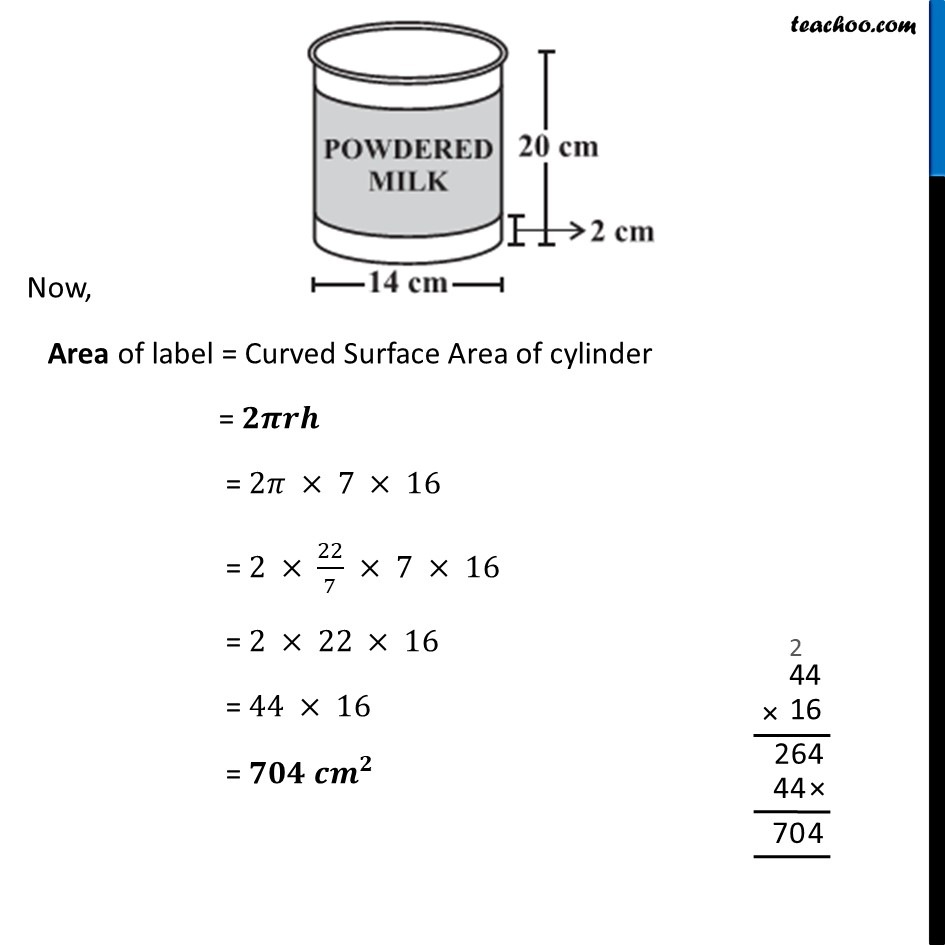Ex 9.2

Chapter 9 Class 8 Mensuration
Serial order wiseLearn in your speed, with individual attention - Teachoo Maths 1-on-1 Class

### Transcript

Ex 9.2, 10 A company packages its milk powder in cylindrical container whose base has a diameter of 14 cm and height 20 cm. Company places a label around the surface of the container (as shown in the figure). If the label is placed 2 cm from top and bottom, what is the area of the label.Here, the label is a hollow cylinder, with Diameter = 14 cm Radius = 14/2 = 7 cm and Height = 20 – 2 – 2 = 16 cm Now, Area of label = Curved Surface Area of cylinder = 𝟐𝝅𝒓𝒉 = 2𝜋 × 7 × 16 = 2 × 22/7 × 7 × 16 = 2 × 22 × 16 = 44 × 16 = 𝟕𝟎𝟒 𝒄𝒎^𝟐